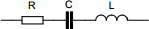# Capacitor impedance calculator

## Calculates the impedance of a capacitor at a given frequency

 Example 1: Must calculate the impedance of a 100nF capacitor at 1Mhz. The capacitor has an inductance of 0.67 nanohenries and ESR of 788 milliohms. View example
 Capacitance farads Inductance henrys ESR ohms Frequency hertzYou may use one of the following SI prefix after a value: p=pico, n=nano, u=micro, m=milli, k=kilo, M=mega, G=giga

Fill all the values of the following
• Capacitance - Capacitance of the capacitor
• Inductance - Inductance of the capacitor
• ESR - Equivalent series resistance of the capacitor
• Frequency - Frequency of the circuit